# E-PolyLearning

 26. The Kozney-Carman equation, rewritten in terms of non-dimensional numbers gives (ΔP/pu² ) proportional to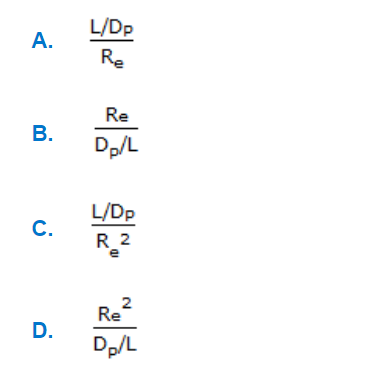a. A b. B c. C d. D

 27. The equation given below is called the __________ .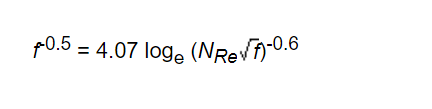a. Colebrook formula b. Von-Karman equation c. Fanning equation d. none of these
 28. Pick out the Blake-Plummer equation (valid for large Nʀₑ) for fluid flow through beds of solids.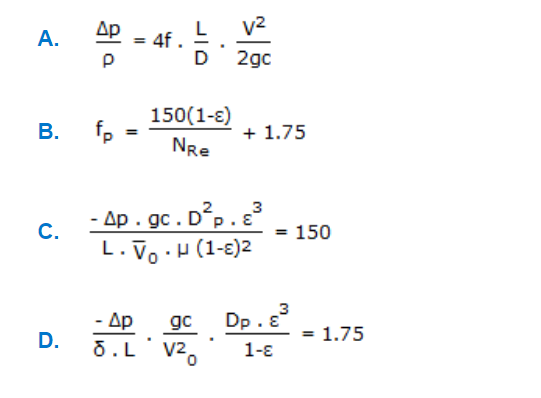a. A b. B c. C d. D
 29. The actual velocity at vena-contracta for flow through an orifice from a reservoir is given by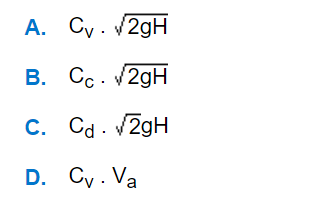a. A b. B c. C d. D
 30. For flow through a venturi at a particular discharge, the correct relationships among heads at points X, Y, and Z are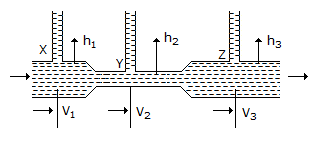a. h₁ > h₂ < h₃ b. h₁ > h₂ > h₃ c. h₂ < h₁ < h₃ d. h₁ < h₂ < h₃